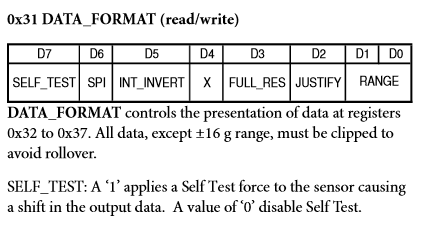# How to read register from datasheet

Hi, I am newbie here

For example I want set SELF_TEST. The SELF_TEST is under DATA_FORMAT (0x31).
In page 12 is table where is D0 | D1 | D2 |...``````Wire.beginTransmission(accel_module);
Wire.write(0x31);
Wire.write( ??? );
Wire.endTransmission();
``````

How can I do this?

Thanks!``````Wire.beginTransmission(accel_module);
Wire.write(0x31);
Wire.write( 0x80 );
Wire.endTransmission();
``````

Can You please explane me why 0x80? Maybe somewhere is manual for this. I googled, but i did not findThe number 80 is in the hexadecimal base, and that is the reason because we add the 0x (the 'x' is from heXadecimal) at the beginning of the number. If you change this number to the binary base you get 1000 0000 (the number is the same but the base is different, the form of representation is different). Each of the 4 bits chunks in binary base are represented by one digit in the hexadecimal base. As you only want the bit D7 (the first from the left) enabled (or set to 1) you only put that to one and all the other to zero. For example, if you want the bit D5 enabled, you will have in binary 0010 0000, that is, in hexadecimal 0x20. Other example, could be if you want the bits D6 and D5 enabled. You will get 0110 0000 in binary, and 0x60 in hexa. Even another example is if you want D7, D5, D1 and D0 enabled. With this you will get 1010 0011 in binary, that is the same that 0xA3 in hexadecimal.

The explanation is very shortened, but I hope you understand.

Yes. Thanks you! This is what I need. Big chocolate cookie from meIf you know your bitwise operators Arduino - Home, you could do:

``````Wire.write(1<<7);
``````

since it says that you want to set bit d7 (the 7 in the bitshift).
I do it for readability and well this way you can map it one to one to the register